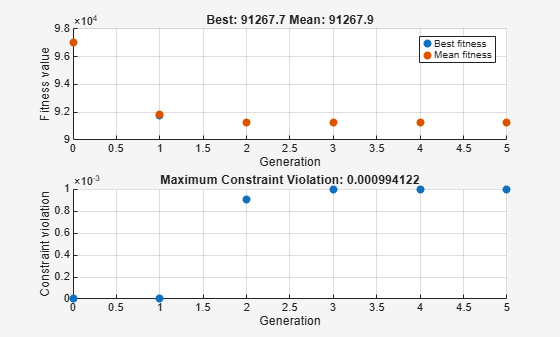Documentation

## Constrained Minimization Using the Genetic Algorithm

This example shows how to minimize an objective function subject to nonlinear inequality constraints and bounds using the Genetic Algorithm.

### Constrained Minimization Problem

We want to minimize a simple fitness function of two variables `x1` and `x2`

``` min f(x) = 100 * (x1^2 - x2) ^2 + (1 - x1)^2; x ```

such that the following two nonlinear constraints and bounds are satisfied

``` x1*x2 + x1 - x2 + 1.5 <=0, (nonlinear constraint) 10 - x1*x2 <=0, (nonlinear constraint) 0 <= x1 <= 1, and (bound) 0 <= x2 <= 13 (bound) ```

The above fitness function is known as 'cam' as described in L.C.W. Dixon and G.P. Szego (eds.), Towards Global Optimisation 2, North-Holland, Amsterdam, 1978.

### Coding the Fitness Function

We create a MATLAB file named `simple_fitness.m` with the following code in it:

``` function y = simple_fitness(x) y = 100 * (x(1)^2 - x(2)) ^2 + (1 - x(1))^2; ```

The Genetic Algorithm function `ga` assumes the fitness function will take one input `x` where `x` has as many elements as number of variables in the problem. The fitness function computes the value of the function and returns that scalar value in its one return argument `y`.

### Coding the Constraint Function

We create a MATLAB file named `simple_constraint.m` with the following code in it:

``` function [c, ceq] = simple_constraint(x) c = [1.5 + x(1)*x(2) + x(1) - x(2); -x(1)*x(2) + 10]; ceq = []; ```

The `ga` function assumes the constraint function will take one input `x` where `x` has as many elements as number of variables in the problem. The constraint function computes the values of all the inequality and equality constraints and returns two vectors `c` and `ceq` respectively.

### Minimizing Using `ga`

To minimize our fitness function using the `ga` function, we need to pass in a function handle to the fitness function as well as specifying the number of variables as the second argument. Lower and upper bounds are provided as `LB` and `UB` respectively. In addition, we also need to pass in a function handle to the nonlinear constraint function.

```ObjectiveFunction = @simple_fitness; nvars = 2; % Number of variables LB = [0 0]; % Lower bound UB = [1 13]; % Upper bound ConstraintFunction = @simple_constraint; [x,fval] = ga(ObjectiveFunction,nvars,[],[],[],[],LB,UB, ... ConstraintFunction)```
```Optimization terminated: average change in the fitness value less than options.FunctionTolerance and constraint violation is less than options.ConstraintTolerance. ```
```x = 1×2 0.8122 12.3104 ```
```fval = 1.3574e+04 ```

Note that for our constrained minimization problem, the `ga` function changed the mutation function to `mutationadaptfeasible`. The default mutation function, `mutationgaussian`, is only appropriate for unconstrained minimization problems.

### `ga` Operators for Constrained Minimization

The `ga` solver handles linear constraints and bounds differently from nonlinear constraints. All the linear constraints and bounds are satisfied throughout the optimization. However, `ga` may not satisfy all the nonlinear constraints at every generation. If `ga` converges to a solution, the nonlinear constraints will be satisfied at that solution.

`ga` uses the mutation and crossover functions to produce new individuals at every generation. The way the `ga` satisfies the linear and bound constraints is to use mutation and crossover functions that only generate feasible points. For example, in the previous call to `ga`, the default mutation function `mutationgaussian` will not satisfy the linear constraints and so the `mutationadaptfeasible` is used instead. If you provide a custom mutation function, this custom function must only generate points that are feasible with respect to the linear and bound constraints. All the crossover functions in the toolbox generate points that satisfy the linear constraints and bounds.

We specify `mutationadaptfeasible` as the `MutationFcn` for our minimization problem by creating options with the `optimoptions` function.

```options = optimoptions(@ga,'MutationFcn',@mutationadaptfeasible); % Next we run the GA solver. [x,fval] = ga(ObjectiveFunction,nvars,[],[],[],[],LB,UB, ... ConstraintFunction,options)```
```Optimization terminated: average change in the fitness value less than options.FunctionTolerance and constraint violation is less than options.ConstraintTolerance. ```
```x = 1×2 0.8122 12.3103 ```
```fval = 1.3573e+04 ```

Next we use `optimoptions` to select two plot functions. The first plot function is `gaplotbestf`, which plots the best and mean score of the population at every generation. The second plot function is `gaplotmaxconstr`, which plots the maximum constraint violation of nonlinear constraints at every generation. We can also visualize the progress of the algorithm by displaying information to the command window using the `Display` option.

```options = optimoptions(options,'PlotFcn',{@gaplotbestf,@gaplotmaxconstr}, ... 'Display','iter'); % Next we run the GA solver. [x,fval] = ga(ObjectiveFunction,nvars,[],[],[],[],LB,UB, ... ConstraintFunction,options)`````` Best Max Stall Generation Func-count f(x) Constraint Generations 1 2524 13579.8 2.1e-07 0 2 4986 13578.2 1.686e-05 0 3 7918 14031 0 0 4 17371 13573.5 0.0009928 0 Optimization terminated: average change in the fitness value less than options.FunctionTolerance and constraint violation is less than options.ConstraintTolerance. ```
```x = 1×2 0.8122 12.3103 ```
```fval = 1.3574e+04 ```

### Providing a Start Point

A start point for the minimization can be provided to `ga` function by specifying the `InitialPopulationMatrix` option. The `ga` function will use the first individual from `InitialPopulationMatrix` as a start point for a constrained minimization. Refer to the documentation for a description of specifying an initial population to `ga`.

```X0 = [0.5 0.5]; % Start point (row vector) options.InitialPopulationMatrix = X0; % Next we run the GA solver. [x,fval] = ga(ObjectiveFunction,nvars,[],[],[],[],LB,UB, ... ConstraintFunction,options)`````` Best Max Stall Generation Func-count f(x) Constraint Generations 1 2520 13578.1 0.000524 0 2 4982 13578.2 1.024e-05 0 3 7914 14030.5 0 0 4 17379 13708.4 0.0001674 0 Optimization terminated: average change in the fitness value less than options.FunctionTolerance and constraint violation is less than options.ConstraintTolerance. ```
```x = 1×2 0.8089 12.3626 ```
```fval = 1.3708e+04 ```

Watch now